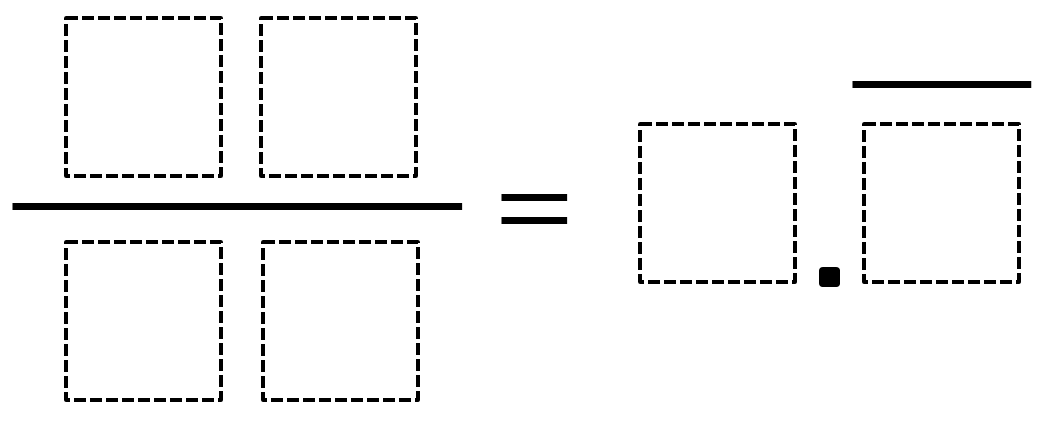# Converting Fractions to Repeating Decimals

Directions: Using the digits 0 to 9, at most one time each, fill in the boxes so that the fraction equals the repeating decimal.### Hint

What does a repeating decimal mean?

There are many possible answers that could work such as

16/48=0.3 (3 is repeated)

84/27=3.1 (1 is repeated)

38/09=4.2 (2 is repeated)

49/63=0.7 (7 is repeated)

68/09=7.5 (5 is repeated)

48/72=0.6 (6 is repeated)

Source: Daniel Luevanos

## Complimentary and Supplementary Angles 2

Directions: Using the digits 0 to 9 at most one time each, fill in the …

1.38/09 = 4.2 repeating

2.49/63 = 0.7 repeating

•68/09 = 7.5 repeating

•48/72 = .6 repeating

•These are all great solutions thanks Phil. I have added them to the answers section of the problem

3.11/12=0.916 repeating

4.5.6.